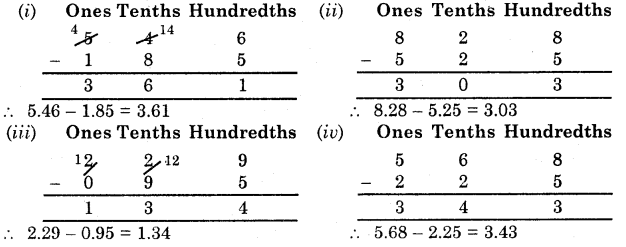# HBSE 6th Class Maths Solutions Chapter 8 Decimals Intext Questions

Haryana State Board HBSE 6th Class Maths Solutions Chapter 14 Practical Geometry Ex 14.5 Textbook Exercise Questions and Answers.

## 14.5

Try These (Page 211) :

Question 1.
Can you now write the following numbers as decimals ?

 Hundreds Tens Ones Tenths (100) (10) (1) (1/10) (i) 5 3 8 1 (ii) 2 7 3 4 (iii) 3 5 4 6

Solution:
(i) 538.1,
(ii) 273.4,
(iii) 354.6.

Question 2.
Write the lengths of Ravi’s and Raju’s pencils in cm using decimals.
Solution:
Length of Ravi’s pencil
= 7 cm 5 mm = 7.5 cm.
Length of Raju’s pencil
= 8 cm 3 mm = 8.3 cm.Question 3.
Make three more examples similar to the one given in question 1 and solve them.
Solution:
(i) Ram has rupees 40 and seventy-five paise and Shyam has rupees 70 and fifty paise. Express these amounts in decimal fractions.
(ii) The length of a wire is 6 metre and 30 cm. Write this length of wire in metres.
(iii) Pallavi and Ashu measured the lengths of their pencils. Pallavi’s pencil was 6 cm and 4 mm long and Ashu’s pencil was 5 cm 7 mm. Write the lengths of their pencils in cm.
Solution:
(i) Rs. 40 and seventy five paise
= Rs. 40.75
Rs. 70 and fifty paise
= Rs. 70.50

(ii) The length of the wire
= 6 metre and 30 cm = 6.30 m.

(iii) Length of Pallavi’s pencil
= 6 cm and 4 mm = 6.4 cm.
Length of Ashu’s pencil
= 5 cm and 7 mm = 5.7 cm.

Try These (Page 212):

Question.
Represent the following numbers on the number line :
(i) 0.6, (ii) 1.2, (Hi) 2.3.
Solution:
(i) We know that 0.6 is more than zero but less than one. There are 6 tenths in it. Divide the unit length between 0 and 1 into 10 equal parts and take 6 parts as shown below: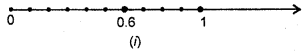(ii) We know that 1.2 is more than one but less than two. There are 2 lengths in it. Divide the unit length between 1 and 2 into 10 the equal parts and take 2 parts as shown below :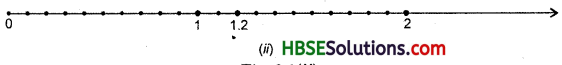(iii) We know that 1.2 is more than one but less than two. There are 2 lengths in it. Divide the unit length between 1 and 2 into 10 the equal parts and take 2 parts as shown below :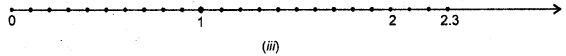Try These (Page 213) :

Question.
Write $$\frac{3}{2}, \frac{4}{5}, \frac{8}{5}$$ in decimal notation.
Solution: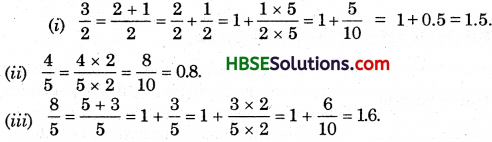Decimals as Fractions :
Write a decimal number 1.2 as a fraction.
Solution:
1.2 = 1.2 $$=\frac{10}{10}+\frac{2}{10}=\frac{10+2}{10}=\frac{12}{10}=\frac{6}{5}$$

Try These

Question 1.
David was measuring the length of his room. He found that the length of his room is 4 m and 25 cm. He wanted to write the length in metres. Can you help him.
Solution:
4 m and 25 cm = 4 + $$\frac{25}{100}$$ = 4.25 m.

Question 2.
Fill in the blanks :
Ordinary fraction Decimal fraction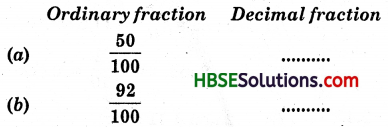Solution:
(a) 0.50,
(b) 0.92.Question 3.
Write the number in decimal fraction: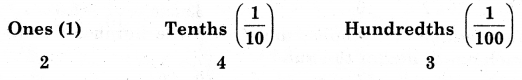Solution:
The number is 2 + $$\frac{4}{10}+\frac{3}{100}$$ = 2 + 0.4 + 0.03 = 2.43.

Try These (Page 224) :

Question.
Compare :
(i) 1.82 and 1.823
(ii) 5.7 and 4.9
(iii) 6.05 and 6.50
(iv) 3.15 and 3.18.
Solution: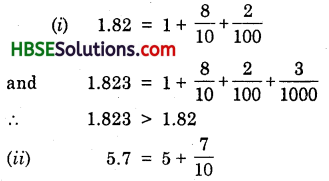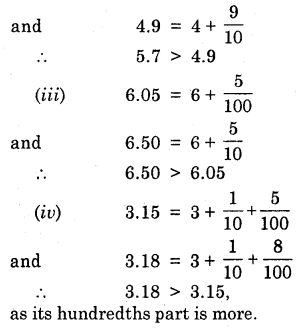Try These (Page 225):

(i) Write 2 rupees 5 paise and 2 rupees 50 paise in decimals.
(it) Write 20 rupees 7 paise and 21 rupees 75 paise in decimals.
Solution:
(i) We know that
100 paise = 1 Re
.’. 1 paise = $$\frac{1}{100}$$ Re = 0.01 Re
.’. 5 paise = $$\frac{5}{100}$$ Re = 0.05 Re
Hence, 2 rupees 5 paise 2.05 Rs.
and 50 paise = $$\frac{50}{100}$$ = 0.5 Re
Hence, 2 rupees 50 paise = 2.50 Rs.
(ii) We know that
100 paise = 1 Re.
1 paise = $$\frac{1}{100}$$ Re = 0.01 Re
7 paise = $$\frac{7}{100}$$ = 0.07 Re
Hence, 20 rupees 7 paise = 20.07 Rs. 75
75 paise = $$\frac{75}{100}$$ = 0.75 Re.
Hence, 21 rupees 75 paise = Rs. 21.75.Try These (Page 226) :

(1) Can you write 4 mm in cm using decimals ?
(2) How will you write 7 cm 5 mm in cm using decimals ?
Solution:
(1) 4mm = $$\frac{4}{10}$$ cm = 0.4 cm
(2) 7 cm 5 mm = 7 + [atex]\frac{5}{10}[/latex] = 7.5 cm.

Try These (Page 227):

Question.
(a) Can you write 456 g as kg using decimals ?
(b) How will you write 2 kg 9 g in kg using decimals ?
Solution:
(a) We know that
1000 g = 1 kg.
1 kg = $$\frac{1}{1000}$$ kg = 0.001 kg
Hence, 456 g = $$\frac{456}{1000}$$ = 0.456 kg.

(b) We know that
1000 g = 1 kg.
1 kg = $$\frac{1}{1000}$$ kg = 0.001 kg
Hence 2 kg 9 g = 2 + $$\frac{9}{1000}$$
= 2 + 0.009
= 2.009 kg.

Try These (Page 229):

Find: (i) 0.29 + 0.36
(ii) 0.7 + 0.08
(iii) 1.54 + 1.80
(iv) 2.66 + 1.85.
Solution: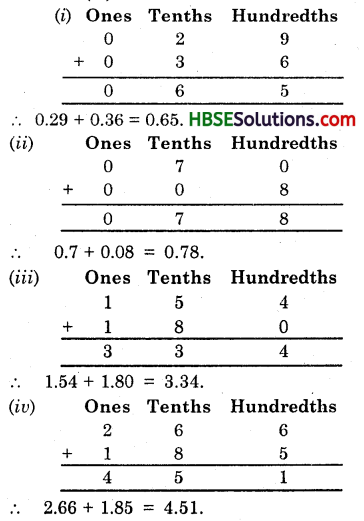Try These {Page 232):

(i) Subtract 1.85 from 5.46
(ii) Subtract 5.25 from 8.28
(iii) Subtract 0.95 from 2.29
(iv) Subtract 2.25 from 5.68.
Solution: